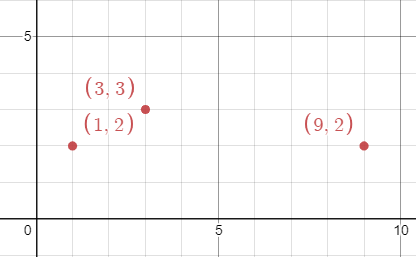##### Welcome to Subscribe On Youtube

Formatted question description: https://leetcode.ca/all/1956.html

# 1956. Minimum Time For K Virus Variants to Spread

Hard

## Description

There are n unique virus variants in an infinite 2D grid. You are given a 2D array points, where points[i] = [x_i, y_i] represents a virus originating at (x_i, y_i) on day 0. Note that it is possible for multiple virus variants to originate at the same point.

Every day, each cell infected with a virus variant will spread the virus to all neighboring points in the four cardinal directions (i.e. up, down, left, and right). If a cell has multiple variants, all the variants will spread without interfering with each other.

Given an integer k, return the minimum integer number of days for any point to contain at least k of the unique virus variants.

Example 1:Input: points = [[1,1],[6,1]], k = 2

Output: 3

Explanation: On day 3, points (3,1) and (4,1) will contain both virus variants.

Example 2:Input: points = [[3,3],[1,2],[9,2]], k = 2

Output: 2

Explanation: On day 2, points (1,3), (2,3), (2,2), and (3,2) will contain the first two viruses.

Example 3:Input: points = [[3,3],[1,2],[9,2]], k = 3

Output: 4

Explanation: On day 4, the point (5,2) will contain all 3 viruses.

Constraints:

• n == points.length
• 2 <= n <= 50
• points[i].length == 2
• 1 <= x_i, y_i <= 10^9
• 2 <= k <= n

## Solution

Use binary search. For a specific number of days, calculate whether there exists a point that contains at least k virus variants.

• class Solution {
public int minDayskVariants(int[][] points, int k) {
int low = 0, high = 1000000000;
while (low < high) {
int mid = (high - low) / 2 + low;
if (containAtLeastK(points, k, mid))
high = mid;
else
low = mid + 1;
}
return low;
}

public boolean containAtLeastK(int[][] points, int k, int days) {
Map<Long, Map<Long, Integer>> lines = new HashMap<Long, Map<Long, Integer>>();
for (int[] point : points) {
int x = point, y = point;
long xLeft = x, yLeft = y - days;
long xBottom = x - days, yBottom = y;
long xRight = x + days, yRight = y;
long xTop = x, yTop = y + days;
long key11 = xLeft + yLeft, key12 = yLeft - xLeft;
Map<Long, Integer> map1 = lines.getOrDefault(key11, new HashMap<Long, Integer>());
map1.put(key12, map1.getOrDefault(key12, 0) + 1);
lines.put(key11, map1);
long key21 = xBottom + yBottom, key22 = yBottom - xBottom + 1;
Map<Long, Integer> map2 = lines.getOrDefault(key21, new HashMap<Long, Integer>());
map2.put(key22, map2.getOrDefault(key22, 0) - 1);
lines.put(key21, map2);
long key31 = xRight + yRight + 1, key32 = yRight - xRight;
Map<Long, Integer> map3 = lines.getOrDefault(key31, new HashMap<Long, Integer>());
map3.put(key32, map3.getOrDefault(key32, 0) - 1);
lines.put(key31, map3);
long key41 = xTop + yTop + 1, key42 = yTop - xTop + 1;
Map<Long, Integer> map4 = lines.getOrDefault(key41, new HashMap<Long, Integer>());
map4.put(key42, map4.getOrDefault(key42, 0) + 1);
lines.put(key41, map4);
}
Map<Long, Integer> ranges = new HashMap<Long, Integer>();
TreeSet<Long> set1 = new TreeSet<Long>(lines.keySet());
for (long key1 : set1) {
Map<Long, Integer> map = lines.get(key1);
TreeSet<Long> set2 = new TreeSet<Long>(map.keySet());
for (long key2 : set2) {
int count = map.get(key2);
ranges.put(key2, ranges.getOrDefault(key2, 0) + count);
}
int total = 0;
TreeSet<Long> rangeSet = new TreeSet<Long>(ranges.keySet());
for (long num : rangeSet) {
int count = ranges.get(num);
total += count;
if (total >= k)
return true;
}
}
return false;
}
}